# 🐢 B4XTurtle - Examples for teachers and parents

#### Erel

##### B4X founder
Staff member
Longtime User
Lists together with custom types make a powerful combination: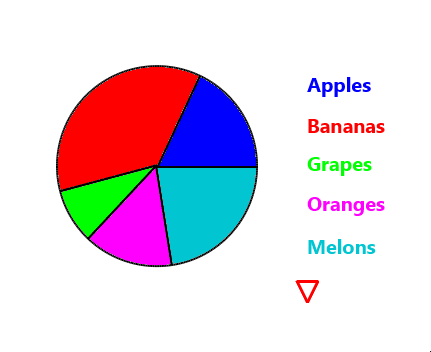A custom type defines a simple object with all kinds of fields. (It is a bit like a class without code, though it can do things that cannot be done with classes.)

Instead of making a list with the values directly we now make a list with more information.
First we define the custom type, this is done in Process_Globals:
B4X:
``Type PieItem (Value As Float, Name As String, Clr As Int)``
Each item stores a value, a name and a color.

We can let the IDE generate a Create sub:There is nothing special about the generated sub. You can modify it as you need.
Adding elements using the CreatePieItem sub:
B4X:
``````Items.Add(CreatePieItem(100, "Apples", xui.Color_Blue))

Now when we iterate over the items we iterate over PieItems:
B4X:
``````For Each pi As PieItem In Items
TotalValues = TotalValues + pi.value
Next``````
This allows us to later draw the items names:
B4X:
``````For Each pi As PieItem In Items
Turtle.SetPenColor(pi.Clr).DrawText(pi.Name).MoveForward(40)
Next``````

Code: (don't forget to add the Type declaration to Process_Globals)
B4X:
``````Sub Turtle_Start
Turtle.SetSpeedFactor(3).SetPenColor(xui.Color_Black)
Turtle.SetX(Turtle.Width / 2 - 80)
Dim Items As List
Items.Initialize
DrawPie(Items)
DrawLabels(Items)
End Sub

Sub DrawLabels (Items As List)
Turtle.PenUp.MoveForward(150)
Turtle.SetFontAndAlignment(xui.CreateDefaultBoldFont(20), "LEFT")
Turtle.SetAngle(90).MoveBackward(80)
For Each pi As PieItem In Items
Turtle.SetPenColor(pi.Clr).DrawText(pi.Name).MoveForward(40)
Next
Turtle.PenDown
End Sub

Sub DrawPie (Items As List)
Dim radius As Float = 100
Dim TotalValues As Float
'For Each - best option when we don't need the index
For Each pi As PieItem In Items
TotalValues = TotalValues + pi.value
Next
Dim TotalAngles As Float
'regular For Next
For i = 0 To Items.Size - 1 'first time index is always 0
Dim pi As PieItem = Items.Get(i)
Dim angle As Float = Floor(pi.value / TotalValues * 360)
If i = Items.Size - 1 Then
'to avoid rounding errors the last element completes the circle.
angle = Ceil(360 - TotalAngles)
End If
TotalAngles = TotalAngles + angle
Next
End Sub

Sub DrawSlice (angle As Float, radius As Float, clr As Int)
For a = 1 To angle
Turtle.MoveForward(2 * cPI * radius / 360).TurnLeft(1)
Next
Turtle.TurnLeft(90).MoveForward(100).TurnLeft(180).PenUp
End Sub

Public Sub CreatePieItem (Value As Float, Name As String, Clr As Int) As PieItem
Dim t1 As PieItem
t1.Initialize
t1.Value = Value
t1.Name = Name
t1.Clr = Clr
Return t1
End Sub``````

••maXim, Swissmade, MarcoRome and 1 other person

#### Erel

##### B4X founder
Staff member
Longtime User

Step #1: Add a reference to XUI Views library - a cross platform library with custom views.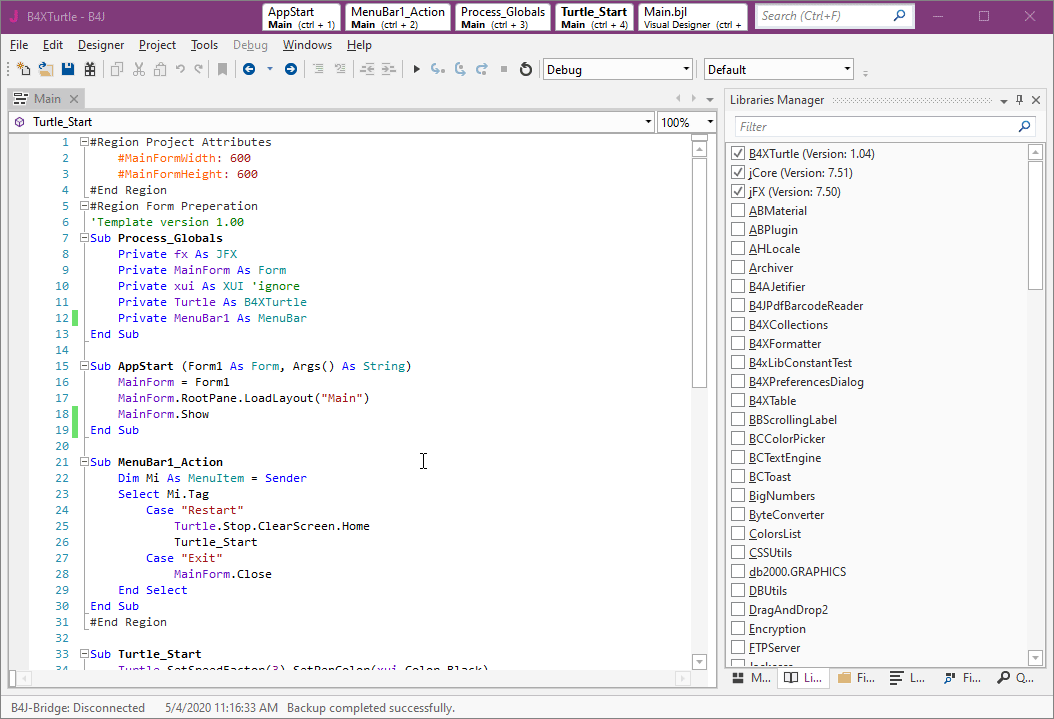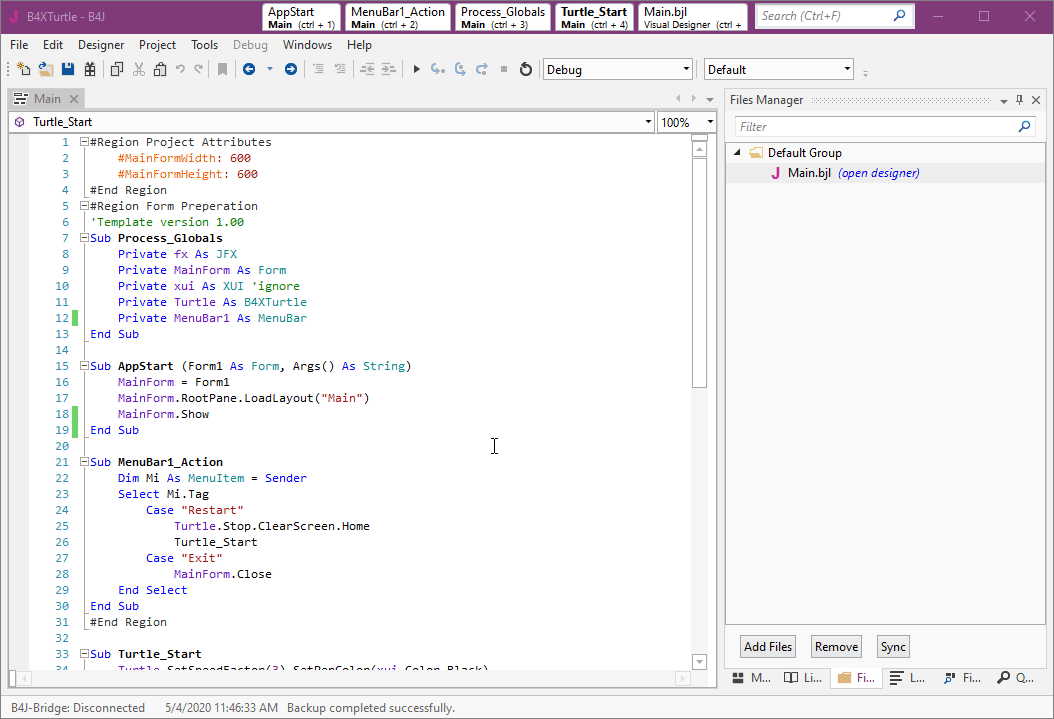Code:
B4X:
``````Sub Turtle_Start
Turtle.SetSpeedFactor(3).SetPenColor(xui.Color_Black)
End Sub

Sub txtNumberOfEdges_EnterPressed
Turtle.ClearScreen.Home
Dim NumberOfEdges As Int = txtNumberOfEdges.Text
For i = 1 To NumberOfEdges
Turtle.MoveForward(100).TurnLeft(360 / NumberOfEdges)
Next
End Sub``````

And the result is: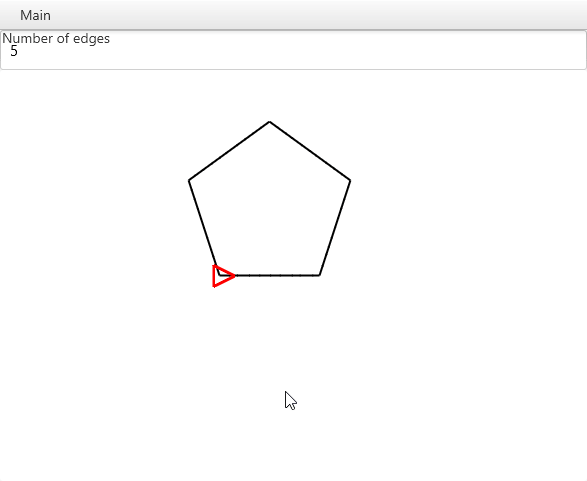Note that if we enter an invalid number the program will crash.
The solution is to verify that the text is actually a number:
B4X:
``````Sub txtNumberOfEdges_EnterPressed
If IsNumber(txtNumberOfEdges.Text) = False Then
xui.MsgboxAsync("Invalid number", "")
Return
End If
Turtle.ClearScreen.Home
Dim NumberOfEdges As Int = txtNumberOfEdges.Text
For i = 1 To NumberOfEdges
Turtle.MoveForward(100).TurnLeft(360 / NumberOfEdges)
Next
End Sub``````

#### Erel

##### B4X founder
Staff member
Longtime UserXUI Views library also includes a cross platform dialog implementation with several templates. In this small example we will use the color template to let the user choose a color and then we will draw a circle with this color.

B4X:
``````Private Dialog As B4XDialog
Private ColorTemplate As B4XColorTemplate``````

2.
B4X:
``````Sub Turtle_Start
If Dialog.IsInitialized = False Then
Dialog.Initialize(MainForm.RootPane) 'Activity in B4A and Page.RootPanel in B4i
Dialog.Title = "🐢 B4XTurtle"
ColorTemplate.Initialize
End If
Turtle.SetSpeedFactor(3).SetPenColor(xui.Color_Black)
End Sub

Sub Turtle_Touch (Args As TurtleTouchArgs)
If Args.Down Then
Wait For (Dialog.ShowTemplate(ColorTemplate, "Ok", "", "Cancel")) Complete (Result As Int)
If Result = xui.DialogResponse_Positive Then
Turtle.SetX(Args.X).SetY(Args.Y)
Turtle.SetPenColor(ColorTemplate.SelectedColor).Arc(360, 50).Fill
End If
End If
End Sub``````

Like most objects in B4X, the dialog and the template need to be initialized once.
We handle the Touch event and check if Args.Down is True.
Line #12 calls the Wait For keyword. Any sub where Wait For or Sleep are called is a 'resumable sub'. This is an important feature of B4X. It is fully explained here: https://www.b4x.com/android/forum/threads/78601/#content

It allows us, the developers, to treat asynchronous tasks as if they were (almost) synchronous.

Once the dialog is closed we check the result. It will be xui.DialogResponse_Positive or xui.DialogResponse_Negative or xui.DialogResponse_Cancel.
If it is positive (OK) we set the pen color and draw a circle.

•maXim

#### Erel

##### B4X founder
Staff member
Longtime User
Tic-Tac-Toe game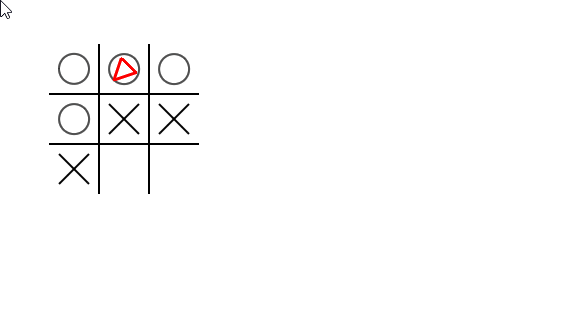For the first time in these examples, we are using an array and it is a two dimension array. Array is the "primitive" version of list, however it can have multiple dimensions which is a useful feature in this case.
The array is used to store the state of each of the 3x3 cells. The value will be one of the constants: CELL_EMPTY, CELL_X or CELL_Y. The constants are declared in the globals sub:
B4X:
``Private Const CELL_EMPTY = 0, CELL_X = 1, CELL_O = 2 As Int``
The Const modifier means that their values cannot be changed.

B4X:
``````    Private Const CELL_EMPTY = 0, CELL_X = 1, CELL_O = 2 As Int
Private CellSize As Int = 50
Private Offset As Int = 50
Private CurrentTurn As Int
Private NumberOfTurns As Int``````

2. Code:
B4X:
``````Sub Turtle_Start
Dim Cells(3, 3) As Int 'all cells will be 0 which happens to be CELL_EMPTY
NumberOfTurns = 0
Turtle.SetSpeedFactor(3).SetPenColor(xui.Color_Black)
DrawBackground
CurrentTurn = CELL_O
End Sub

Sub DrawBackground
Turtle.ClearScreen
For i = 1 To 2
Turtle.SetX(Offset + i * CellSize).SetY(Offset).SetAngle(90)
Turtle.MoveForward(CellSize * 3)
Turtle.SetX(Offset).SetY(Offset + i * CellSize).SetAngle(0)
Turtle.MoveForward(CellSize * 3)
Next
End Sub

Sub Turtle_Touch (Args As TurtleTouchArgs)
If Args.Down Then
If NumberOfTurns >= 9 Then Return
Dim x As Int= (Args.X - Offset) / CellSize
Dim y As Int = (Args.Y - Offset) / CellSize
Log(x & ", " & y)
If x < 0 Or y < 0 Or x > 2 Or y > 2 Then Return
If Cells(x, y) <> CELL_EMPTY Then Return 'already marked
Cells(x, y) = CurrentTurn
NumberOfTurns = NumberOfTurns + 1
DrawCell(x, y)
CheckEndGame
If CurrentTurn = CELL_X Then CurrentTurn = CELL_O Else CurrentTurn = CELL_X
End If
End Sub

Sub DrawCell (X As Int, Y As Int)
If Cells(X, Y) = CELL_O Then
Turtle.SetX(Offset + x * CellSize + CellSize / 2).SetY(Offset + y * CellSize + CellSize / 2)
Turtle.Arc(360, CellSize / 2 - 10)
Else
Turtle.SetX(Offset + x * CellSize + 10).SetY(Offset + y * CellSize + 10)
Turtle.MoveTo(Offset + x * CellSize + CellSize - 10, Offset + y * CellSize + CellSize - 10)
Turtle.SetX(Offset + x * CellSize + CellSize - 10).SetY(Offset + y * CellSize + 10)
Turtle.MoveTo(Offset + x * CellSize + 10, Offset + y * CellSize + CellSize - 10)
End If
End Sub

Sub CheckEndGame
'The computer is fast enough and we can easily check all possible cases every step.
Dim winner As Int = CELL_EMPTY
'rows
For y = 0 To 2
Dim FirstCell As Int = Cells(0, y)
If FirstCell = CELL_EMPTY Then Continue 'continue with the next row
If Cells(1, y) = FirstCell And Cells(2, y) = FirstCell Then
winner = FirstCell
Exit
End If
Next
'columns
For x = 0 To 2
Dim FirstCell As Int = Cells(x, 0)
If FirstCell = CELL_EMPTY Then Continue
If Cells(x, 1) = FirstCell And Cells(x, 2) = FirstCell Then
winner = FirstCell
Exit
End If
Next
'diagonals
If Cells(0, 0) <> CELL_EMPTY Then
FirstCell = Cells(0, 0)
If Cells(1, 1) = FirstCell And Cells(2, 2) = FirstCell Then
winner = FirstCell
End If
End If
If Cells(0, 2) <> CELL_EMPTY Then
FirstCell = Cells(0, 2)
If Cells(1, 1) = FirstCell And Cells(2, 0) = FirstCell Then
winner = FirstCell
End If
End If
'do we have a winner?
If winner <> CELL_EMPTY Then
Dim s As String = "The winner is: "
If winner = CELL_O Then s = s & "O" Else s = s & "X"
s = s & "!!!"
xui.MsgboxAsync(s, "B4XTurtle")
NumberOfTurns = 9 '<--- don't allow more turns
Else If NumberOfTurns = 9 Then
xui.MsgboxAsync("No one won :(", "B4XTurtle")
End If
End Sub``````

Last edited:

#### Erel

##### B4X founder
Staff member
Longtime User
Strings

All real programs need to deal with strings. What is a string? A string is a static sequence of characters.
A string literal is a sequence of characters enclosed with quotes:
B4X:
``Dim s As String = "abc 123 ,./"``
In B4X there is another type of string literal called smart string literal: [B4X] Smart String Literal

The '&' character is the concatenation operator. It joins two strings and return a new string.

There are many important aspects to strings. This example demonstrates several of the built-in methods: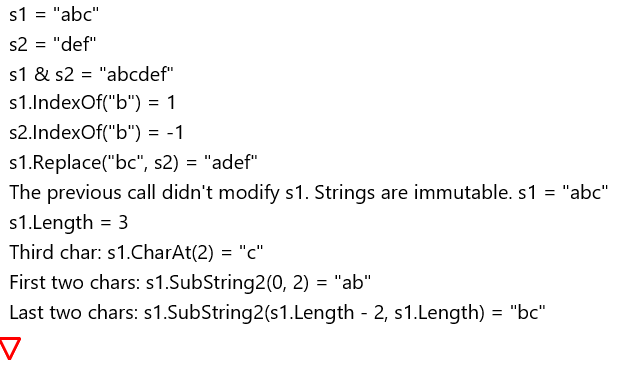B4X:
``````Sub Turtle_Start
Turtle.SetFontAndAlignment(xui.CreateDefaultFont(20), "LEFT").PenUp.SetY(20)
Dim s1 As String = "abc"
Dim s2 As String = "def"
Print(\$"s1 = "\${s1}""\$) 'this is a smart string literal. No need to escape quotes and it supports interpolation. It can also be multiline.
Print(\$"s2 = "\${s2}""\$)
Print(\$"s1 & s2 = "\${s1 & s2}""\$)
Print(\$"s1.IndexOf("b") = \${s1.IndexOf("b")}"\$)
Print(\$"s2.IndexOf("b") = \${s2.IndexOf("b")}"\$)
Print(\$"s1.Replace("bc", s2) = "\${s1.Replace("bc", s2)}""\$)
Print(\$"The previous call didn't modify s1. Strings are immutable. s1 = "\${s1}""\$)
Print(\$"s1.Length = \${s1.Length}"\$)
Print(\$"Third char: s1.CharAt(2) = "\${s1.CharAt(2)}""\$)
Print(\$"First two chars: s1.SubString2(0, 2) = "\${s1.SubString2(0, 2)}""\$)
Print(\$"Last two chars: s1.SubString2(s1.Length - 2, s1.Length) = "\${s1.SubString2(s1.Length - 2, s1.Length)}""\$)
End Sub

Sub Print(s As String)
Turtle.SetX(10).DrawText(s).SetAngle(90).MoveForward(30)
End Sub``````

•maXim and Johan Hormaza

#### Erel

##### B4X founder
Staff member
Longtime User
Control the turtle with the keys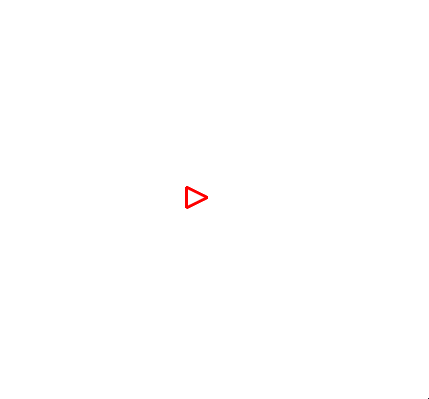This is a B4J example that uses jGameViewHelper library to intercept the keys.

1. Add a reference to jGameViewHelper
B4X:
``````Sub gvh_KeyPressed (KeyCode As String) As Boolean
If KeyCode = "Up" Then Up = True
If KeyCode = "Down" Then Down = True
If KeyCode = "Left" Then Left = True
If KeyCode = "Right" Then Right = True
If KeyCode = "Space" And Space = False Then
PenColor = Turtle.RandomColor
PenSize = Rnd(1, 20)
Space = True
End If
Return False
End Sub``````
The keys state is stored in several global variables. This state is then applied in the timer tick event.

2. Complete code:
B4X:
``````#Region Form Preperation
'Template version 1.00
Sub Process_Globals
Private fx As JFX
Private MainForm As Form
Private xui As XUI 'ignore
Private Turtle As B4XTurtle
Private gvh As GameViewHelper
Private Up, Right, Left, Down, Space As Boolean
Private Timer1 As Timer
Private PenColor As Int
Private PenSize As Int
End Sub

Sub AppStart (Form1 As Form, Args() As String)
MainForm = Form1
MainForm.Show
Timer1.Initialize("Timer1", 30)
End Sub

Dim Mi As MenuItem = Sender
Select Mi.Tag
Case "Restart"
Turtle.Stop.ClearScreen.Home
Turtle_Start
Case "Exit"
MainForm.Close
End Select
End Sub
#End Region

Sub gvh_KeyPressed (KeyCode As String) As Boolean
If KeyCode = "Up" Then Up = True
If KeyCode = "Down" Then Down = True
If KeyCode = "Left" Then Left = True
If KeyCode = "Right" Then Right = True
If KeyCode = "Space" And Space = False Then
PenColor = Turtle.RandomColor
PenSize = Rnd(1, 20)
Space = True
End If
Return False
End Sub

Sub gvh_KeyReleased (KeyCode As String) As Boolean
If KeyCode = "Up" Then Up = False
If KeyCode = "Down" Then Down = False
If KeyCode = "Left" Then Left = False
If KeyCode = "Right" Then Right = False
If KeyCode = "Space" Then Space = False
Return False
End Sub

Sub Timer1_Tick
Turtle.Stop 'try it without this and see what happens
If Space Then
Turtle.PenDown.SetPenColor(PenColor).SetPenSize(PenSize)
Else
Turtle.PenUp
End If
If Left Then
Turtle.TurnLeft(10)
Else If Right Then
Turtle.TurnRight(10)
End If
If Up Then
Turtle.MoveForward(30)
Else If Down Then
Turtle.MoveBackward(30)
End If

End Sub

Sub Turtle_Start
Turtle.DegreesPerSecond = 10000
Turtle.SetFontAndAlignment(xui.CreateDefaultFont(20), "LEFT")
Timer1.Enabled = True
End Sub``````

••maXim, Swissmade, Johan Hormaza and 3 others

#### Erel

##### B4X founder
Staff member
Longtime User
Another flower:B4X:
``````Sub Turtle_Start
Turtle.SetSpeedFactor(3).SetPenColor(xui.Color_Blue).SetPenSize(3)
For i = 1 To 10
Leaf
Turtle.TurnLeft(36).SetPenColor(Turtle.RandomColor)
Next
End Sub

Sub Leaf
For i1 = 1 To 2
For i2 = 1 To 80
Turtle.MoveForward(2).TurnRight(1)
Next
Turtle.TurnRight(100)
Next
End Sub``````

You can play with the leaf length and thickness. The total turns in the Leaf sub should reach 360.

#### Erel

##### B4X founder
Staff member
Longtime User
Lets fill the flower:Each leaf is drawn on a different layer. Otherwise the overlapping regions will not be filled properly.

B4X:
``````Sub Turtle_Start
Turtle.SetSpeedFactor(3).SetPenColor(xui.Color_Blue).SetPenSize(3).SetNumberOfLayers(11)
For i = 1 To 10
Turtle.SetCurrentLayer(i - 1) 'first one is 0
Leaf
Turtle.TurnLeft(36).SetPenColor(Turtle.RandomColor)
Next
Turtle.SetCurrentLayer(30)
Turtle.SetPenColor(xui.Color_Yellow).Arc(360, 20).Fill.SetTurtleVisible(False)
End Sub

Sub Leaf
For i1 = 1 To 2
For i2 = 1 To 80
Turtle.MoveForward(2).TurnRight(1)
Next
Turtle.TurnRight(100)
Next
Turtle.PushState
Turtle.TurnRight(20).PenUp.MoveForward(20).Fill
Turtle.PopState
End Sub``````

See post #31 for a nicer implementation based on StartPolygon / FillPolygon.

Last edited:
••maXim, inakigarm, Peter Simpson and 2 others

#### Erel

##### B4X founder
Staff member
Longtime User
Rainbow drawingsColors in B4X are represented as Int numbers. The numbers hold four 0 - 255 values, for the four ARGB channels: Alpha, Red, Green, Blue.
There are however other ways to represent colors. HSV (Hue, Saturation, Value) is another common format. The advantage of HSV is that the channels are more similar to the way humans see colors.
This examples includes code that converts HSV colors to ARGB. This allows us to draw using nice rainbow colors.

The important code is in the touch event. Instead of using Turtle.MoveTo we calculate the angle and distance ourselves. This allows us to change the color every step.

Process Globals:
B4X:
``    Private ColorCounter As Int``

B4X:
``````Sub Turtle_Start
Turtle.RabbitMode
Turtle.SetSpeedFactor(3).SetPenColor(xui.Color_Blue).SetPenSize(8)
End Sub

Sub Turtle_Touch (Args As TurtleTouchArgs)
If Args.Down Then
Turtle.SetX(Args.X).SetY(Args.Y)
Else
Dim dx As Float = Args.X - Turtle.GetX
Dim dy As Float = Args.Y - Turtle.GetY
Dim NewDegree As Float = ATan2D(dy, dx)
Turtle.SetAngle(NewDegree)
For i = 1 To Sqrt(Power(dy, 2) + Power(dx, 2))
Turtle.SetPenColor(HSVToColor(255, ColorCounter, 1, 1)).MoveForward(1)
ColorCounter = ColorCounter + 1
Next
End If
End Sub

Private Sub HSVToColor(alpha As Int, h As Float, s As Float, v As Float) As Int
Dim r, g, b As Float
Dim hi As Float = Floor(h / 60)
Dim hbucket As Int =  hi Mod 6
Dim f As Float = h / 60 - hi
Dim p As Float = v * (1 - s)
Dim q As Float = v  * (1 - f * s)
Dim t As Float = v * (1 - (1 - f) * s)
Select hbucket
Case 0
r = v
g = t
b = p
Case 1
r = q
g = v
b = p
Case 2
r = p
g = v
b = t
Case 3
r = p
g = q
b = v
Case 4
r = t
g = p
b = v
Case 5
r = v
g = p
b = q
End Select
Return xui.Color_ARGB(alpha, Round(r * 255), Round(g * 255), Round(b * 255))
End Sub``````

#### Erel

##### B4X founder
Staff member
Longtime User
Colorful square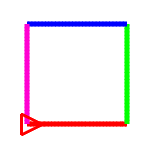We previously saw how we can use a For loop to draw a square without repeating the same code 4 times.

However what if we want to draw each edge with a different (non-random) color?

We can repeat the code several times and change the color:

B4X:
``````Sub Turtle_Start
Turtle.SetPenSize(5)
Turtle.SetPenColor(xui.Color_Red).MoveForward(100).TurnLeft(90)
Turtle.SetPenColor(xui.Color_Green).MoveForward(100).TurnLeft(90)
Turtle.SetPenColor(xui.Color_Blue).MoveForward(100).TurnLeft(90)
Turtle.SetPenColor(xui.Color_Magenta).MoveForward(100).TurnLeft(90)
End Sub``````
While it doesn't look too bad in this small example, such code should be avoided.

Lets see two better options:
1.
B4X:
``````Sub Turtle_Start
Turtle.SetPenSize(5)
Dim colors() As Int = Array As Int(xui.Color_Red, xui.Color_Green, xui.Color_Blue, xui.Color_Magenta)
For i = 0 To colors.Length - 1 'important to start from 0!
Turtle.SetPenColor(colors(i)).MoveForward(100).TurnLeft(90)
Next
End Sub``````

2. And a bit more elegant and also safer:
B4X:
``````Sub Turtle_Start
Turtle.SetPenSize(5)
For Each clr As Int In Array(xui.Color_Red, xui.Color_Green, xui.Color_Blue, xui.Color_Magenta)
Turtle.SetPenColor(clr).MoveForward(100).TurnLeft(90)
Next
End Sub``````

#### Erel

##### B4X founder
Staff member
Longtime User
StartPolygon / FillPolygon

The Fill method has two problems:
- It is a slow method as many pixels need to be tested. This is especially noticeable on mobile devices.
- The result is not perfect due to antialiasing effects.

Starting from B4XTurtle v1.06 there is another way to fill shapes. It solves the above problems.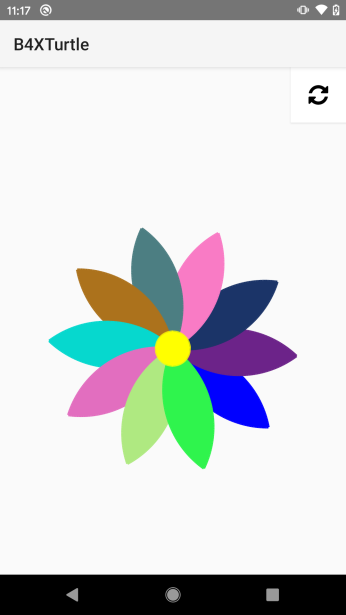First we need to call Turtle.StartPolygon. The turtle will add the current point to the list of points and will start tracking the following points.
Then we call Turtle.FillPolygon to fill the polygon (shape) created by the points.

For example to draw a full square:
B4X:
``````Turtle.StartPolygon
For i = 1 To 4
Turtle.MoveForward(100).TurnRight(90)
Next
Turtle.FillPolygon``````

The list of points is cleared after calling FillPolygon.

Drawing a nice flower:
B4X:
``````Sub Turtle_Start
Turtle.SetSpeedFactor(3).SetPenSize(3)
For i = 1 To 10
Turtle.SetPenColor(Turtle.RandomColor)
Leaf
Turtle.TurnLeft(36)
Next
Turtle.SetPenColor(xui.Color_Yellow)
FullCircle(20)
Turtle.SetTurtleVisible(False)
End Sub

Sub Leaf
Turtle.StartPolygon
For i1 = 1 To 2
For i2 = 1 To 80
Turtle.MoveForward(2).TurnRight(1)
Next
Turtle.TurnRight(100)
Next
Turtle.FillPolygon
End Sub

Turtle.PushState
Turtle.StartPolygon
For i = 1 To 360
Turtle.MoveForward(2 * cPI * Radius / 360).TurnRight(1)
Next
Turtle.FillPolygon
Turtle.PopState
End Sub``````
Note that unlike the previous "flower example" this time we don't need to create multiple layers.

•Johan Hormaza and Peter Simpson

#### Erel

##### B4X founder
Staff member
Longtime User
Fill the screen

B4X:
``````Sub FillScreenWithColor (Clr As Int)
Turtle.PushState.RabbitMode.SetX(0).SetY(0).SetPenColor(Clr)
Turtle.StartPolygon
Turtle.MoveTo(0, Turtle.Height - 1).MoveTo(Turtle.Width - 1, Turtle.Height - 1).MoveTo(Turtle.Width - 1, 0)
Turtle.FillPolygon
Turtle.PopState
End Sub``````

Usage:
B4X:
``FillScreenWithColor (xui.Color_Red)``

#### Erel

##### B4X founder
Staff member
Longtime User
Chess board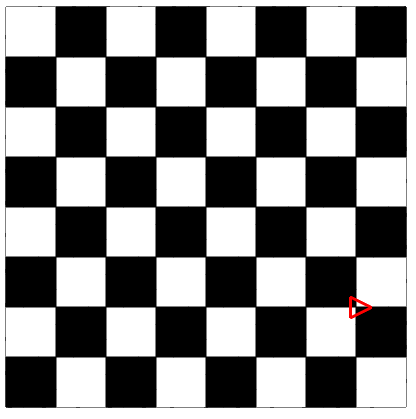B4X:
``````Sub Turtle_Start
Turtle.SetSpeedFactor(10).SetPenColor(xui.Color_Blue).SetPenSize(1)
Dim CellSize As Float = 50
Dim Offset As Float = 10
Turtle.SetX(Offset).SetY(Offset)
FullSquare(CellSize * 8, xui.Color_White, xui.Color_Black)
For x = 0 To 7
For y = 0 To 7
If (x + y) Mod 2 = 1 Then 'black cells
Turtle.SetX(Offset + x * CellSize).SetY(Offset + y * CellSize)
FullSquare(CellSize, xui.Color_Black, xui.Color_Black)
End If
Next
Next
End Sub

Sub FullSquare (EdgeSize As Float, FillColor As Int, StrokeColor As Int)
Turtle.StartPolygon.SetPenColor(StrokeColor)
For i = 1 To 4
Turtle.MoveForward(EdgeSize).TurnRight(90)
Next
Turtle.SetPenColor(FillColor).FillPolygon
End Sub``````

••Johan Hormaza, Peter Simpson and LucaMs

#### Erel

##### B4X founder
Staff member
Longtime User
3D Turtle example by @sorex: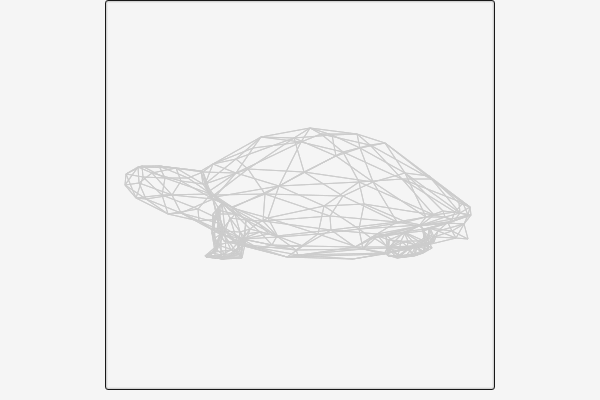#### Attachments

• Turtle3D.zip
9 KB · Views: 326
••Pravee7094, josejad, Johan Hormaza and 2 others

#### Erel

##### B4X founder
Staff member
Longtime User
Please don't post questions here. Only B4XTurtle examples.

#### Chris Salonikas

##### Member
Longtime User
Plotting like a geometric lathe examples - With and Without B4XTurtle
Plotting formulas were obtained from Book "microcomputer art" by Ross Edwards (1985). The book is available in the National Library of Australia.

Plotting like a geometric lathe. | B4X Programming Forum
Refreshing a Layout (clearing a plot in a panel) | B4X Programming Forum

Example 1 B4X without B4XTurtle by @Mahares and @klaus

B4X:
``````Sub Button1_Click
Dim Rect1 As Rect
Rect1.Initialize(0, 0, 100%x, 100%y)
cvsActivity.DrawRect(Rect1, Colors.black, True, 1dip)
Panel1.Invalidate

Private a As Double
Private x,y,r,s,n As Int
Private lastx, lasty As Int

r=Rnd(220, 250)
s=Rnd(50, 100)
n=Rnd(20, 40)

For a = 0 To 2 * cPI+0.01 Step 0.01
x = r * Cos(a) + s * Cos(n * a) + w
y = r * Sin(a) + s * Sin(n * a) + h
If a = 0 Then
lastx = x
lasty = y
End If
If a <= 2.1  Then
cvsActivity.DrawLine(lastx, lasty, x, y, Colors.Yellow, 2dip)
Else if a>2.1 And a < = 4.2 Then
cvsActivity.DrawLine(lastx, lasty, x, y, Colors.Red, 2dip)
Else
cvsActivity.DrawLine(lastx, lasty, x, y, Colors.cyan, 2dip)
End If
Panel1.Invalidate
Sleep(0)  'change to suit
lastx = x
lasty = y
Next
Log(\$"r: \${r},   s: \${s},   n: \${n},"\$)
Log("done")
End Sub``````

r = 275, s = 125, n = 10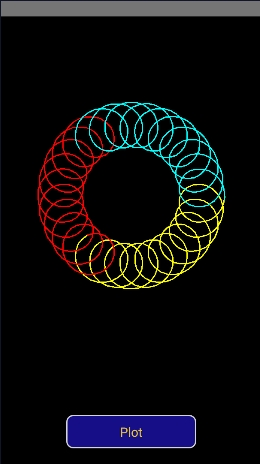#### Attachments

• GeoPlot1.zip
9.6 KB · Views: 182
Last edited:
•Erel

#### Chris Salonikas

##### Member
Longtime User
Plotting like a geometric lathe examples - With and Without B4XTurtle

Example 1 Using B4XTurtle by @Johan Schoeman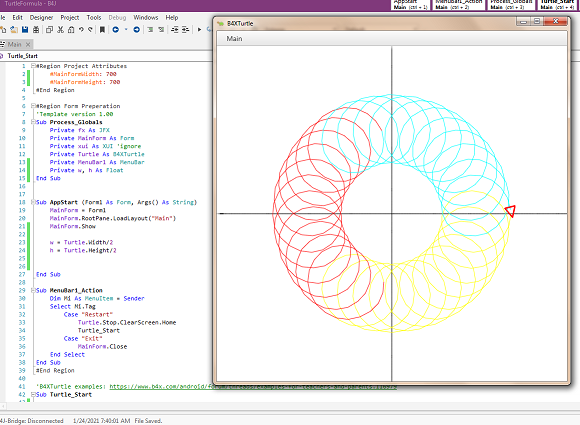#### Attachments

• TurtleFormula1.zip
3.8 KB · Views: 180
•#### Chris Salonikas

##### Member
Longtime User
Plotting like a geometric lathe examples - With and Without B4XTurtle

Example 2 B4X without B4XTurtle by @Mahares and @klaus

B4X:
``````Sub Button1_Click
Dim Rect1 As Rect
Rect1.Initialize(0, 0, 100%x, 100%y)
cvsActivity.DrawRect(Rect1, Colors.black, True, 1dip)
Panel1.Invalidate

Private a As Double
Private x,y,r,s,t,n,m As Int
Private lastx, lasty As Int

'    r=Rnd(275, 351)
'    s=Rnd(130, 210)
'    t=Rnd(40, 120)
'    n=Rnd(-15, -2)
'    m=Rnd(-52, -4)

r = 160
s = 80
t = 40
n = -8
m = -26

For a = 0 To 2 * cPI + 0.01 Step 0.01
x = r * Cos(a) + s * Cos(n * a) + t * Cos(m * a) + w
y = r * Sin(a) + s * Sin(n * a) + t * Sin(m * a) + h
If a = 0 Then
lastx = x
lasty = y
End If
If a <= 2.1 Then
cvsActivity.DrawLine(lastx, lasty, x, y, Colors.Yellow, 2dip)
Else if a>2.1 And a < = 4.2 Then
cvsActivity.DrawLine(lastx, lasty, x, y, Colors.Red, 2dip)
Else
cvsActivity.DrawLine(lastx, lasty, x, y, Colors.cyan, 2dip)
End If
Panel1.Invalidate
Sleep(0)  'change to suit
lastx = x
lasty = y
Next
Log(\$"r: \${r},  s: \${s}, t: \${t},  n: \${n}, m: \${m}, "\$)
Log("done")
End Sub``````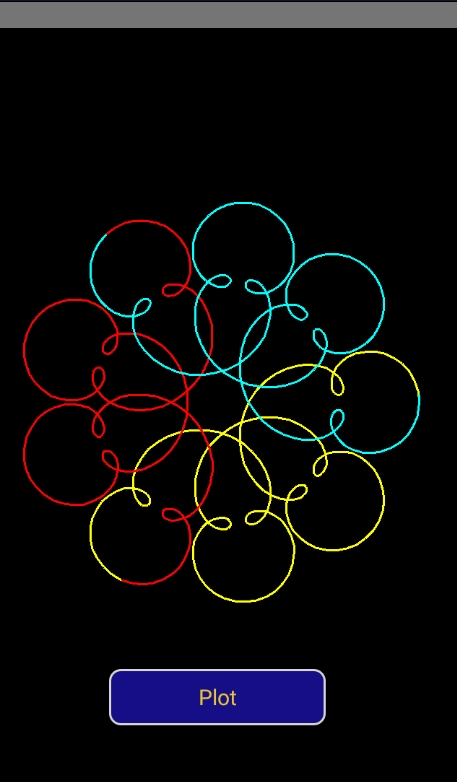#### Attachments

• GeoPlot2.zip
9.6 KB · Views: 175
•Johan Hormaza and Erel

#### Chris Salonikas

##### Member
Longtime User
Plotting like a geometric lathe examples - With and Without B4XTurtle

Example 2 Using B4XTurtle by @Johan Schoeman

r = 160, s = 80, t = 40, m = -26, n = -8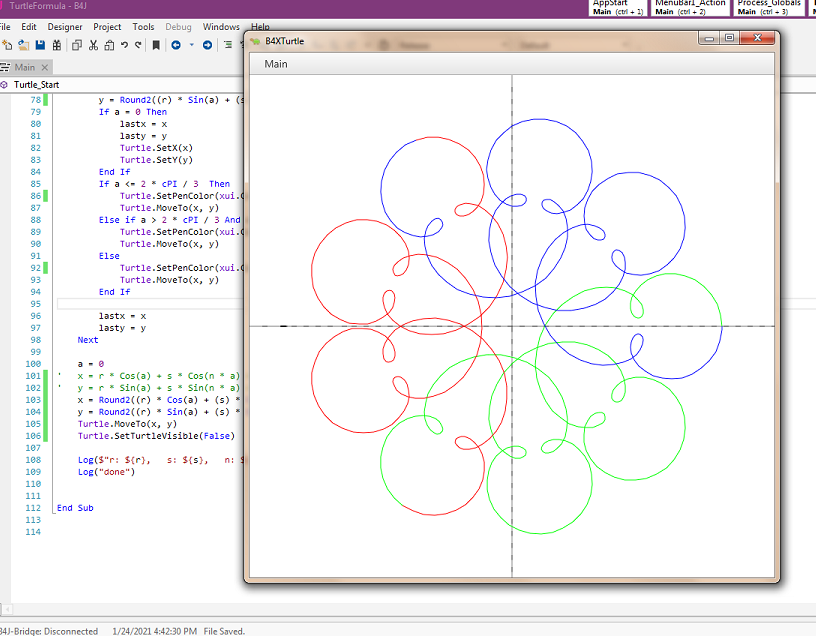B4X:
``````Sub Turtle_Start

Turtle.SetSpeedFactor(5)
Turtle.SetPenSize(0.5)
Turtle.SetPenColor(xui.Color_Black)
Turtle.SetX(0)
Turtle.SetY(h)
Turtle.MoveTo(Turtle.Width, h)

Turtle.SetX(w)
Turtle.SetY(0)
Turtle.MoveTo(w, Turtle.Height)

Turtle.SetPenSize(1)
Dim lastx As Float
Dim lasty As Float
Dim a As Double
'    Dim x, y,r,s,n As Int
lastx = w
lasty = h
'    r=150
'    s=75
'    n=30

Dim x, y, r, s, t, m, n As Int
r = 160
s = 80
t = 40
n = -8
m = -26

For a = 0 To 2 * cPI Step 0.01
'        x = r * Cos(a) + s * Cos(n * a) + w
'        y = r * Sin(a) + s * Sin(n * a) + h
x = Round2((r) * Cos(a) + (s) * Cos(n * a) + (t) * Cos(m * a)+ w, 0)
y = Round2((r) * Sin(a) + (s) * Sin(n * a) + (t) * Sin(m * a)+ h, 0)
If a = 0 Then
lastx = x
lasty = y
Turtle.SetX(x)
Turtle.SetY(y)
End If
If a <= 2 * cPI / 3  Then                              '0 to 1/3 of the way
Turtle.SetPenColor(xui.Color_Green)
Turtle.MoveTo(x, y)
Else if a > 2 * cPI / 3 And a < = 2 * 2 * cPI / 3 Then '1/3 of the way to 2/3's of the way
Turtle.SetPenColor(xui.Color_Red)
Turtle.MoveTo(x, y)
Else
Turtle.SetPenColor(xui.Color_Blue)                 '2/3's of the way up to the end
Turtle.MoveTo(x, y)
End If

lastx = x
lasty = y
Next

a = 0
'    x = r * Cos(a) + s * Cos(n * a) + w
'    y = r * Sin(a) + s * Sin(n * a) + h
x = Round2((r) * Cos(a) + (s) * Cos(n * a) + (t) * Cos(m * a)+ w, 0)
y = Round2((r) * Sin(a) + (s) * Sin(n * a) + (t) * Sin(m * a)+ h, 0)
Turtle.MoveTo(x, y)
Turtle.SetTurtleVisible(False)

Log(\$"r: \${r},   s: \${s},   n: \${n},"\$)
Log("done")

End Sub``````

•Johan Hormaza and Erel

#### Johan Schoeman

##### Expert
Longtime User
Sin & Cos

B4X:
``````#Region Project Attributes
#MainFormWidth: 650
#MainFormHeight: 650
#End Region

#Region Form Preperation
'Template version 1.00
Sub Process_Globals
Private fx As JFX
Private MainForm As Form
Private xui As XUI 'ignore
Private Turtle As B4XTurtle
Dim zerox, zeroy, offset As Float

End Sub

Sub AppStart (Form1 As Form, Args() As String)
MainForm = Form1
MainForm.Show
End Sub

#End Region

Sub Turtle_Start
'    Turtle.SetPenColor(xui.Color_Blue).SetPenSize(5)
'    Turtle.MoveForward(100)
offset = 25  'leave a gap of 25 at the top and bottom as well as on the left and right of the X and Y axis
zerox = (Turtle.Width - 2 * offset ) / 2
zeroy = (Turtle.Height - 2 * offset) / 2

Dim amplitude As Int = 2

Turtle.SetSpeedFactor(5)
Turtle.mBase.Color = xui.Color_LightGray                  'set the Turtle background color
Turtle.PenUp
Turtle.SetX(zerox + offset)
Turtle.SetY(0 + offset)
Turtle.PenDown
Turtle.MoveTo(zerox + offset, 2 * zeroy + offset)          'Draw the y-axis
Turtle.SetX(0 + offset)
Turtle.SetY(zeroy + offset)
Turtle.MoveTo(2 * zerox + offset , zeroy + offset)         'Draw the x-axis

Dim x As Float = 0
Dim y As Float = 0

Turtle.SetPenColor(xui.Color_Blue)
For i = -180 To 180
x = offset + (2 * zerox / (2 * cPI)) * ((i+180)/360) * 2 * cPI            'add the offset to the calculated x position
y = offset + ((Cos(i * 2 * cPI/360) * -1 * (zeroy))/amplitude) + zeroy          'add the offset to the calculated y position

If i = -180 Then
Turtle.SetX(x)
Turtle.SetY(y)
End If
Turtle.MoveTo(x,y)
Next

Turtle.SetPenColor(xui.Color_Yellow)
For i = -180 To 180
x = offset + (2 * zerox / (2 * cPI)) * ((i+180)/360) * 2 * cPI            'add the offset to the calculated x position
y = offset + (Cos(i * 2 * cPI/360) * -1 * (zeroy)) + zeroy          'add the offset to the calculated y position
If i = -180 Then
Turtle.SetX(x)
Turtle.SetY(y)
End If
Turtle.MoveTo(x,y)
Next

Turtle.SetPenColor(xui.Color_Red)
For i = -180 To 180
x = offset + (2 * zerox / (2 * cPI)) * ((i+180)/360) * 2 * cPI            'add the offset to the calculated x position
y = offset + ((Sin(i * 2 * cPI/360) * -1 * (zeroy))/amplitude) + zeroy          'add the offset to the calculated y position
If i = -180 Then
Turtle.SetX(x)
Turtle.SetY(y)
End If
Turtle.MoveTo(x,y)
Next

Turtle.SetPenColor(xui.Color_Cyan)
For i = -180 To 180
x = offset + (2 * zerox / (2 * cPI)) * ((i+180)/360) * 2 * cPI            'add the offset to the calculated x position
y = offset + ((Cos((i + 30) * 2 * cPI/360) * -1 * (zeroy))/amplitude) + zeroy          'add the offset to the calculated y position
If i = -180 Then
Turtle.SetX(x)
Turtle.SetY(y)
End If
Turtle.MoveTo(x,y)
Next

Turtle.SetPenColor(xui.Color_Magenta)
For i = -180 To 180
x = offset + (2 * zerox / (2 * cPI)) * ((i+180)/360) * 2 * cPI            'add the offset to the calculated x position
y = offset + ((Sin((i + 30) * 2 * cPI/360) * -1 * (zeroy))/amplitude) + zeroy          'add the offset to the calculated y position
If i = -180 Then
Turtle.SetX(x)
Turtle.SetY(y)
End If
Turtle.MoveTo(x,y)
Next

End Sub``````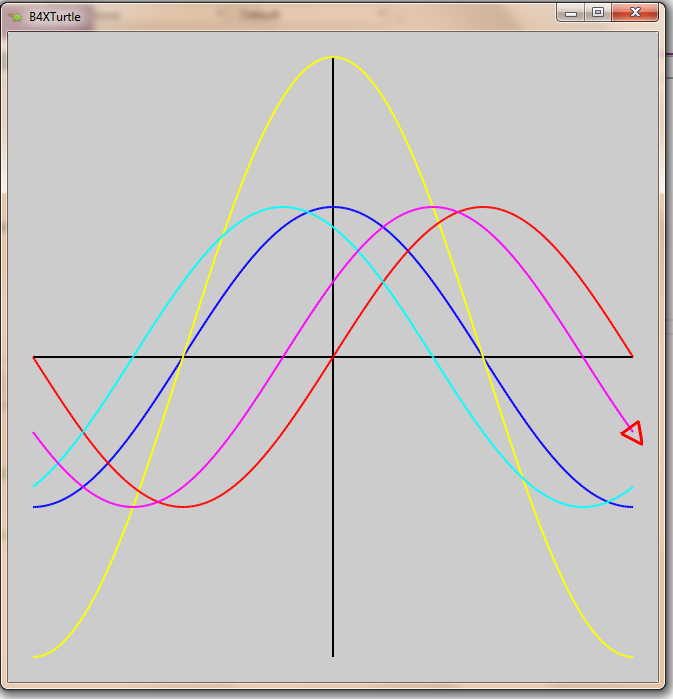#### Attachments

• TurtleSinCos.zip
3.6 KB · Views: 166
•Erel and Johan Hormaza